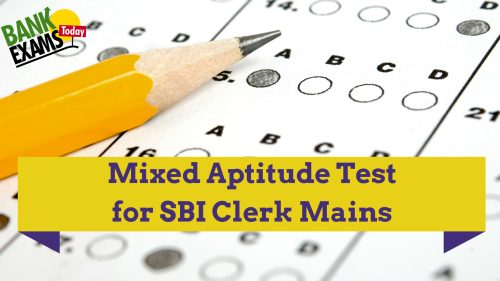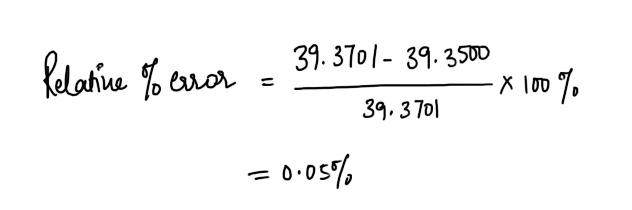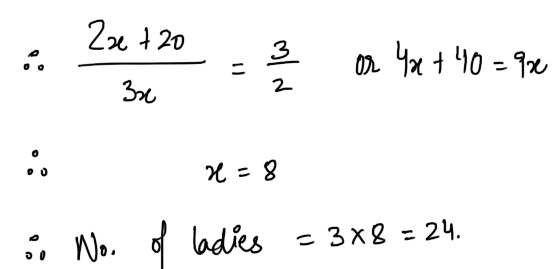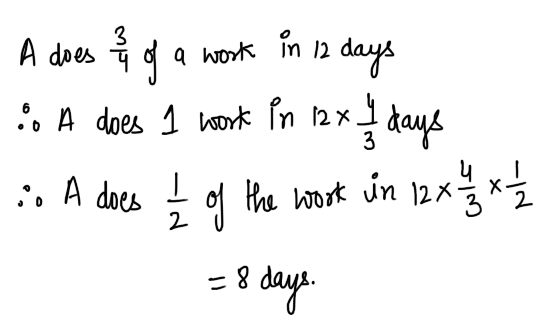# Mixed Aptitude Quiz for SBI Clerk Mains (Part - 11)#### Ques 1.

A metre is taken to be equal to 39.3701 inches. If the value is approximated to 39 7/20 inches, the relative percentage error is
(a) 5%
(b) 0.5%
(c) 0.05%
(d) 0.005%
Ans 1.#### Ques 2.

A number when divided by 169 leaves 78 as remainder. what would be the remainder when the same number is divided by 13 ?
(a) 0
(b) 1
(c) 6
(d) 9
Ans 2. Given number = 169q + 78
= 13 ( 13 q + 6)
=> The number is completely divisible by  13.

#### Ques 3.

Three bells toll at intervals of 36 s, 40 s, 48 s, respectively . They start together . after how many minutes will they next toll together ?
(a) 6
(b) 12
(c) 18
(d) 24
Ans 3.#### Ques 4.

A's salaryis 20% less than B's salary . By how much per cent is B's salary more than A's ?
(a) 16 2/3
(b) 20
(c) 25
(d) 33 1/3
Ans 4.#### Ques 5.

The ratio of the number of gents to ladies in a party was 2:3 . When 20 more gents joined the group, the ratio was reversed . The number of ladies in the party was
(a) 16
(b) 24
(c) 30
(d) 36
Ans 5.  Let the  number  of gents and ladies be 2x  and 3x respectively#### Ques 6.

The average marks in Mathematics for 5 students was found to be 50. Later on, it was discovered that in the case of one student the marks 48 were misread as 84. the correct average is
(a) 40.2
(b) 40.8
(c) 42.8
(d) 48.2
Ans 6.#### Ques 7.

Two trains start from stations A and B travel towards each other at speeds of 50 km/hour and 60 km/hour respectively . At the time of their meeting , the second train has travelled 120 km more than the first. The distance between A and B is
(a) 990 km
(b) 1200 km
(c) 1320 km
(d) 1440 km
Ans 7. Let the distance travelled by the first train be x km.
. . Distance travelled by the second train = x + 120 km
Now,  x/50 = x + 120/60
. . x  = 600 km
Distance between A and B = x + x + 120 = 1200 + 120 = 1320 km

#### Ques 8.

A can do 3/4 of a work 12 days. He can finish 1/2 of the work in
(a) 4
(b) 8
(c) 12
(d) 16
Ans 8.#### Ques 9.

A,B and C are supposed to finish 19/23 of the work together.How much shall be paid to B ?
(a) Rs 82
(b) Rs 92
(c) Rs 300
(d) Rs 437
Ans 9.#### Ques 10.

Three cubes of iron whose sides are 6 cm, 8 cm and 10 cm respectively are melted and formed into a single cube. The edge of the new cube formed is
(a) 12 cm
(b) 14 cm
(c) 16 cm
(d) 18 cm
Ans 10. volume of new cube = volume of cube I + Volume of cube II + Volume of cube  III
or volume of new cube = 6+ 83 + 103
216 + 512 + 1000
or x3 = 1728 = 123
.  .  x = 12 cm.

#### Ques 11.

A wire , bent in the form of a sqaure , encloses an area of 484 cm2 .If the same wire is bent so as to from a circle, then the area enclosed will be
(a) 484 cm2
(b) 538 2/7 cm2
(c) 616 cm2
(d) 644 cm2
Ans 11. ( side)2 = 484
. . side = 22 cm
Length of wire = 22 x 4 = 88 cm
Now, 2r = 88
..  r = 14 cm
. .  Area  enclosed = r x 14 x 14
= 616 cm2.

#### Ques 12.

Two cylindrical pots contain the same amount of water.If their diameter are in the ratio 2 :3 , the ratio of their heights is
(a) 2 : 3
(b) 9 : 2
(c) 9 : 3
(d) 9 : 4
Ans 12.